# RD Sharma Solutions For Class 7 Maths Exercise 21.1 Chapter 21 Mensuration - II (Area of Circle)

Students can view and download the PDF of RD Sharma Solutions for Class 7 Chapter 21 Mensuration – II (Area of Circle) Exercise 21.1 available here. Our specialist teachers formulate solutions to these exercises to help you with your exam preparation to achieve good marks in Maths. The RD Sharma Solutions for Class 7 are stepwise and detailed to make learning easy for students. This exercise deals with the circle. It consists of twenty main questions. Let us have a look at important topics covered in this exercise.

• Definition and meaning of the circle
• Circular region
• Chord of a circle
• Diameter of circle
• Concentric circles
• Congruent circles
• Circumference of circles
• Relation between diameter and circle

## Download the PDF of RD Sharma Solutions For Class 7 Chapter 21 – Mensuration – II (Area of Circle) Exercise 21.1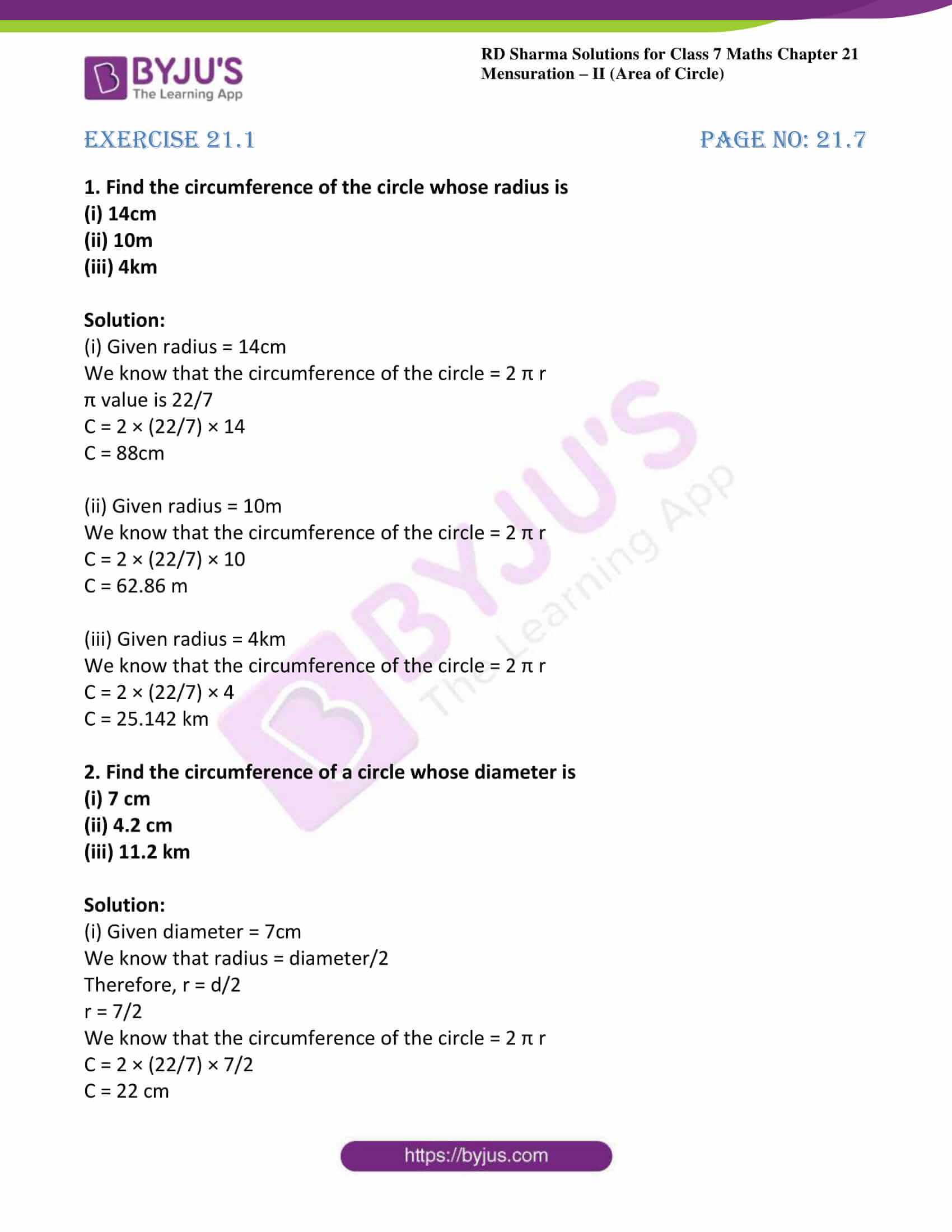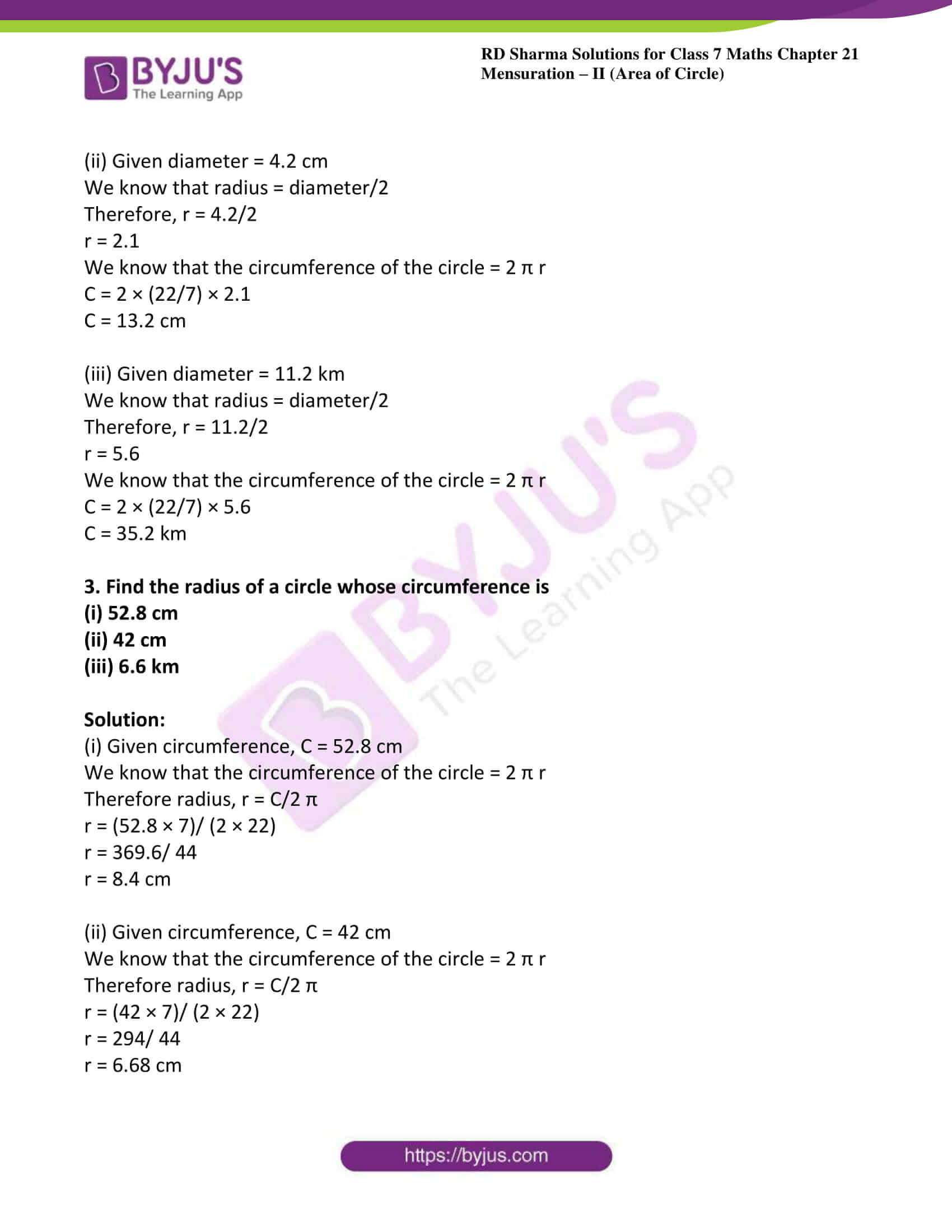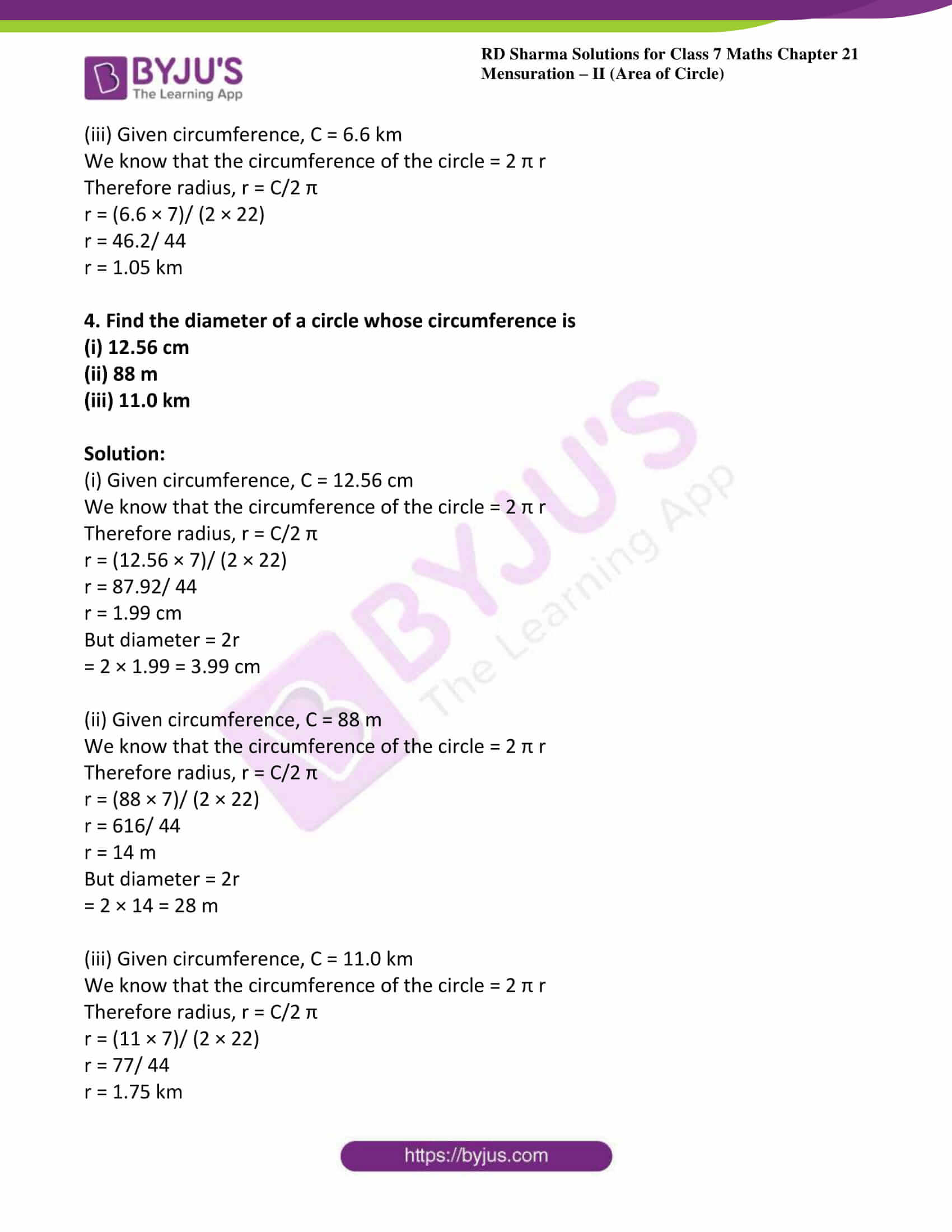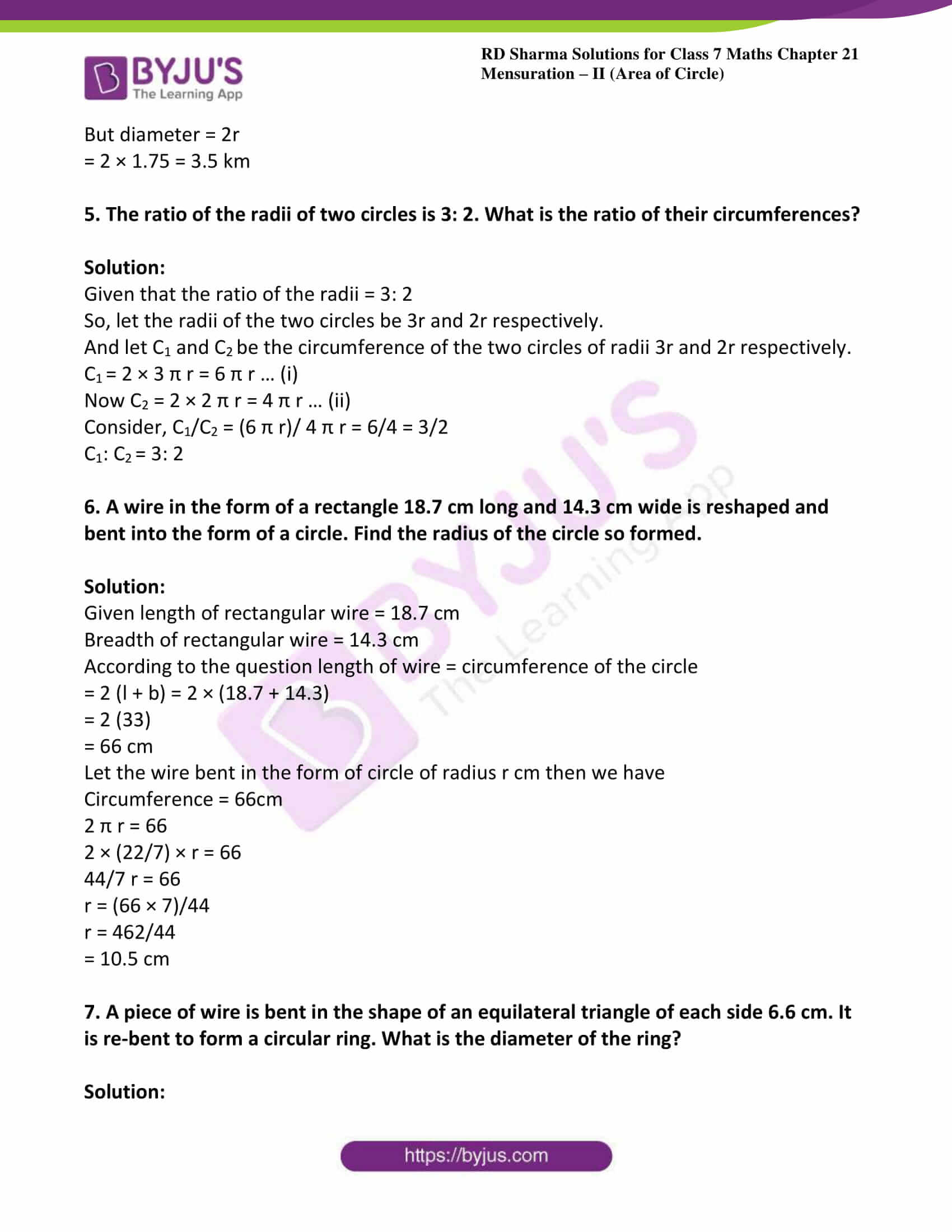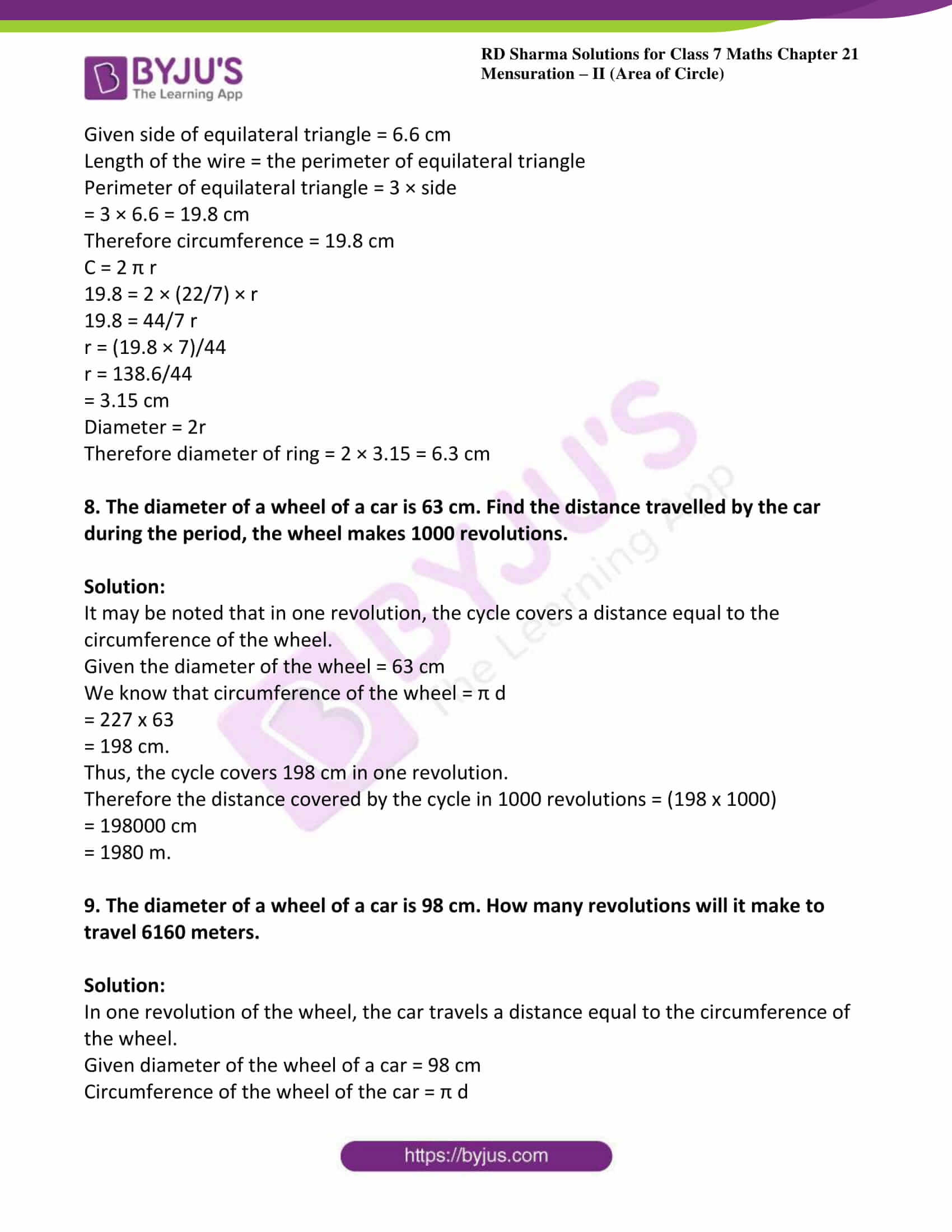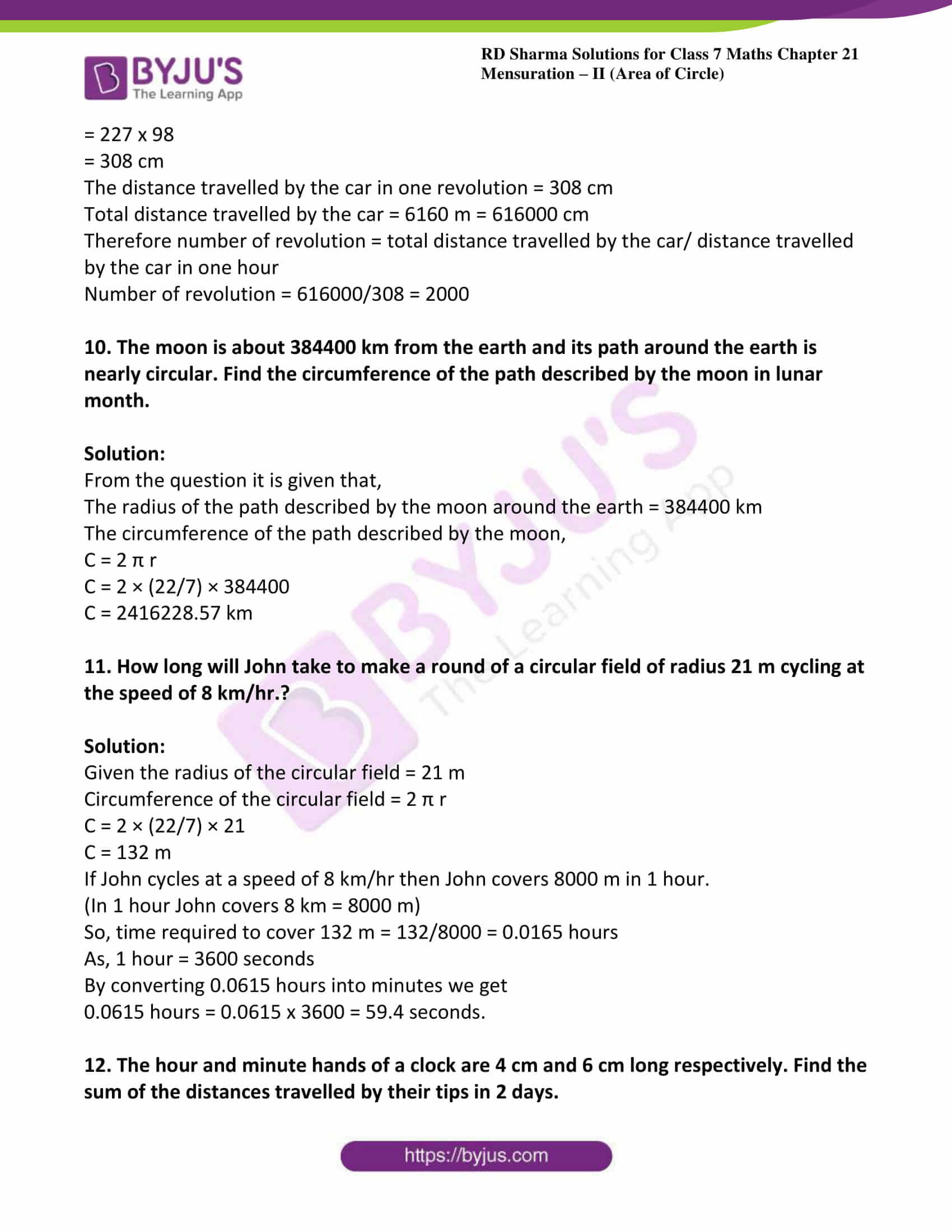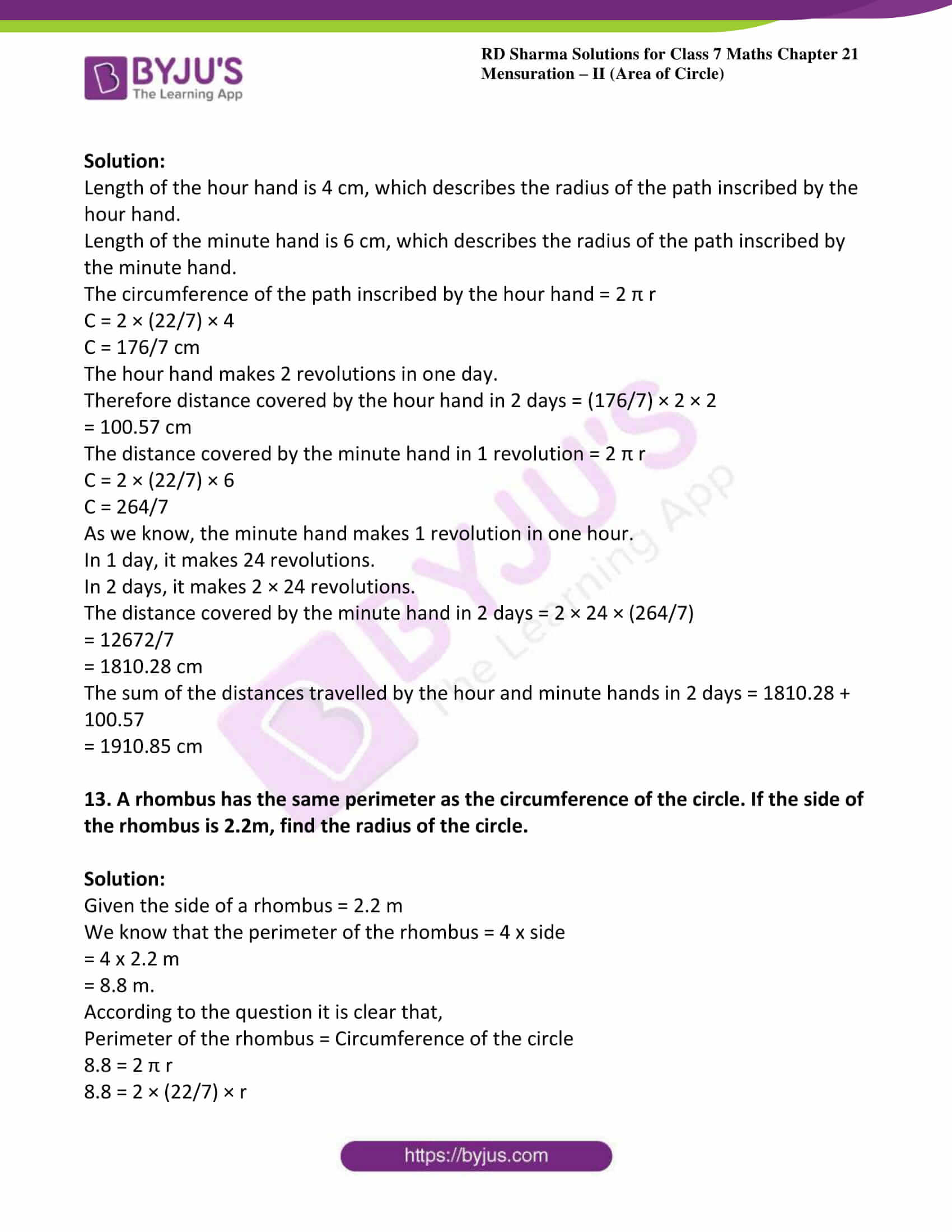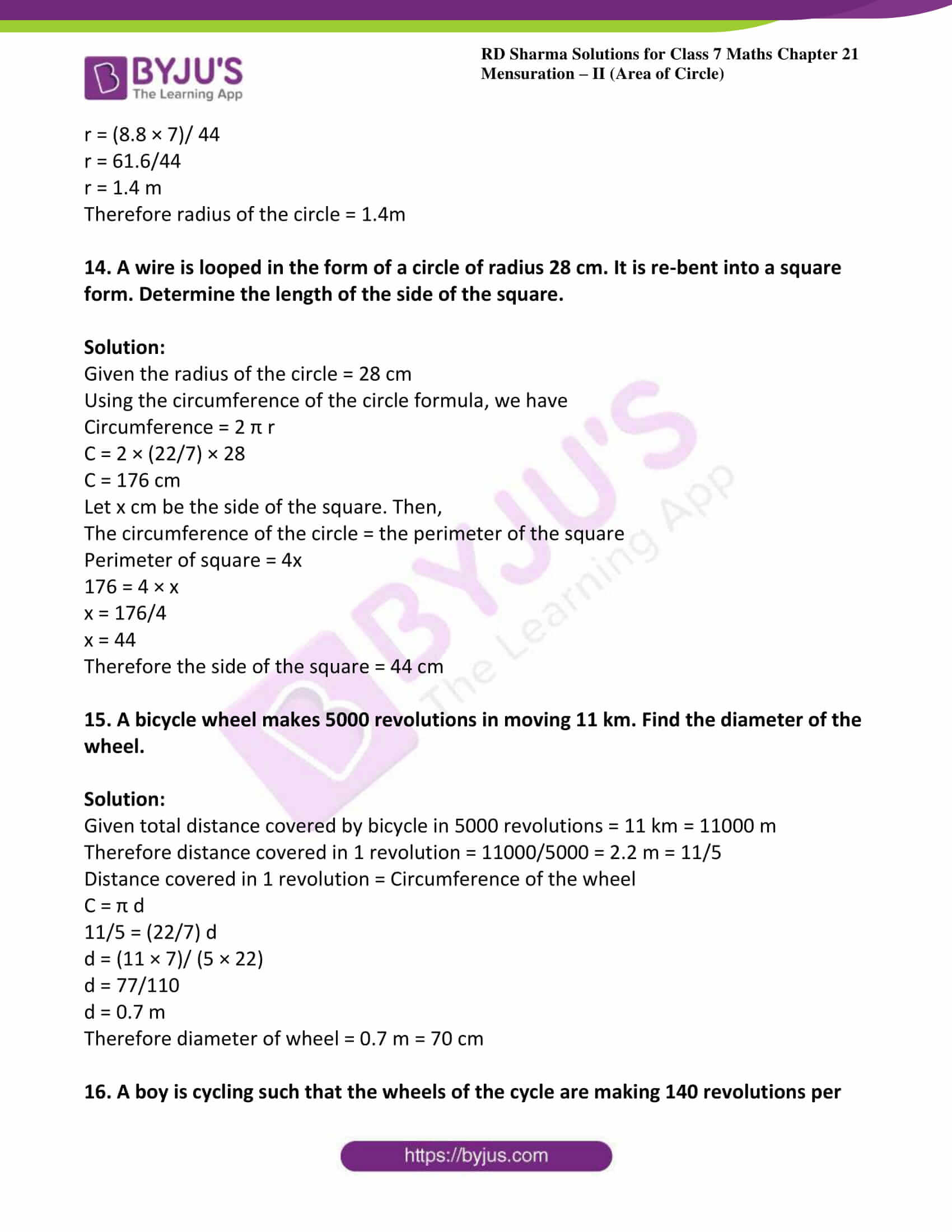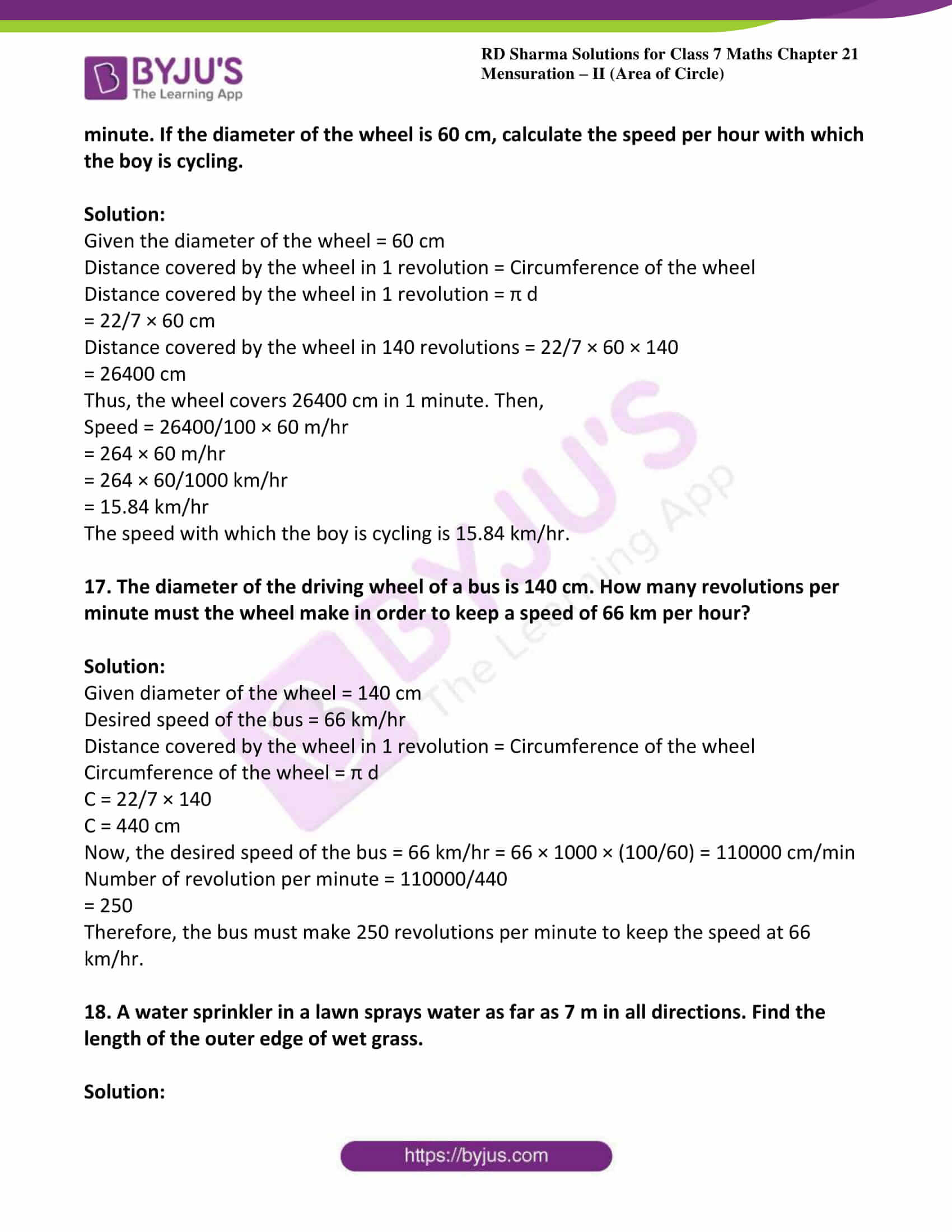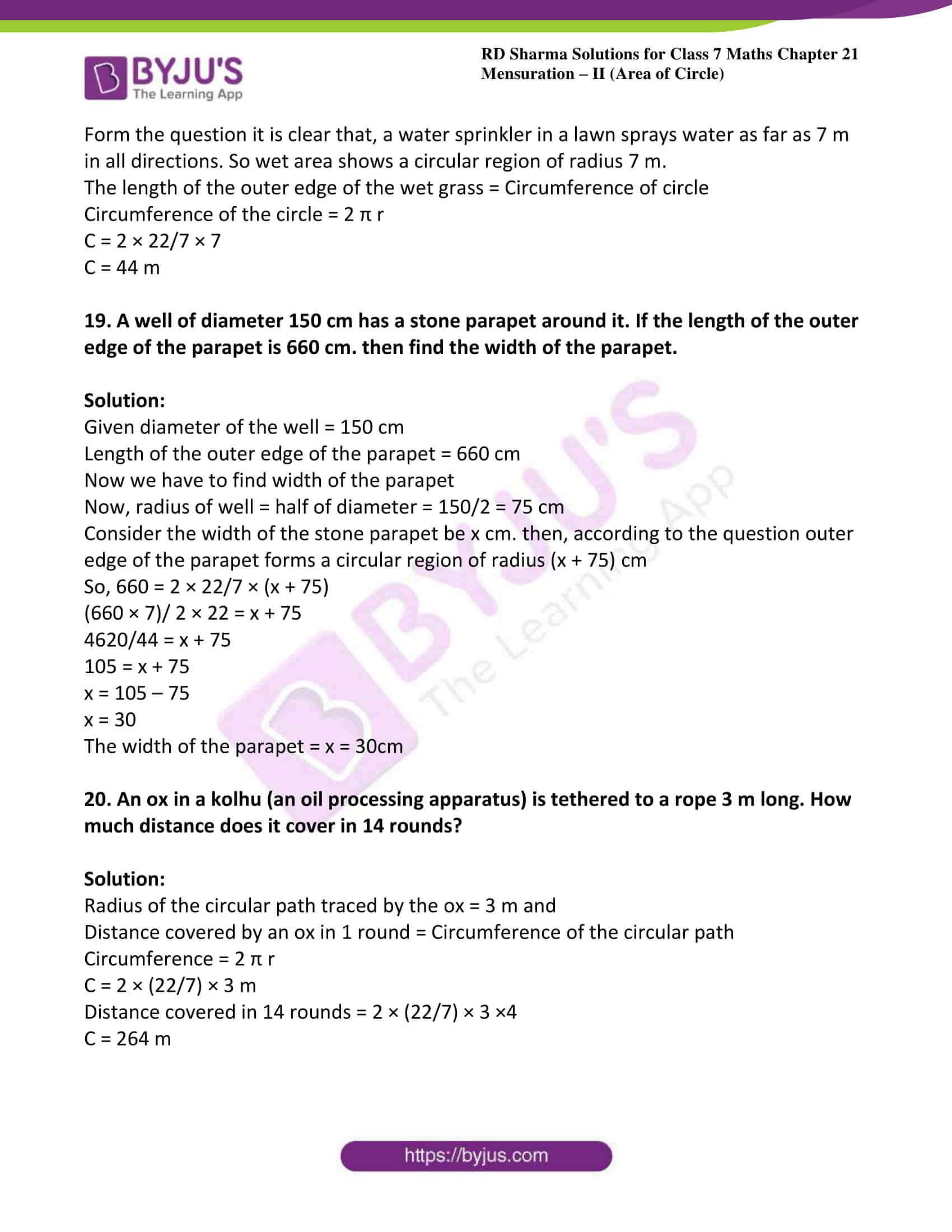### Access answers to Maths RD Sharma Solutions For Class 7 Chapter 21 – Mensuration – II (Area of Circle) Exercise 21.1

1. Find the circumference of the circle whose radius is

(i) 14cm

(ii) 10m

(iii) 4km

Solution:

We know that the circumference of the circle = 2 π r

π value is 22/7

C = 2 × (22/7) × 14

C = 88cm

We know that the circumference of the circle = 2 π r

C = 2 × (22/7) × 10

C = 62.86 m

We know that the circumference of the circle = 2 π r

C = 2 × (22/7) × 4

C = 25.142 km

2. Find the circumference of a circle whose diameter is

(i) 7 cm

(ii) 4.2 cm

(iii) 11.2 km

Solution:

(i) Given diameter = 7cm

We know that radius = diameter/2

Therefore, r = d/2

r = 7/2

We know that the circumference of the circle = 2 π r

C = 2 × (22/7) × 7/2

C = 22 cm

(ii) Given diameter = 4.2 cm

We know that radius = diameter/2

Therefore, r = 4.2/2

r = 2.1

We know that the circumference of the circle = 2 π r

C = 2 × (22/7) × 2.1

C = 13.2 cm

(iii) Given diameter = 11.2 km

We know that radius = diameter/2

Therefore, r = 11.2/2

r = 5.6

We know that the circumference of the circle = 2 π r

C = 2 × (22/7) × 5.6

C = 35.2 km

3. Find the radius of a circle whose circumference is

(i) 52.8 cm

(ii) 42 cm

(iii) 6.6 km

Solution:

(i) Given circumference, C = 52.8 cm

We know that the circumference of the circle = 2 π r

Therefore radius, r = C/2 π

r = (52.8 × 7)/ (2 × 22)

r = 369.6/ 44

r = 8.4 cm

(ii) Given circumference, C = 42 cm

We know that the circumference of the circle = 2 π r

Therefore radius, r = C/2 π

r = (42 × 7)/ (2 × 22)

r = 294/ 44

r = 6.68 cm

(iii) Given circumference, C = 6.6 km

We know that the circumference of the circle = 2 π r

Therefore radius, r = C/2 π

r = (6.6 × 7)/ (2 × 22)

r = 46.2/ 44

r = 1.05 km

4. Find the diameter of a circle whose circumference is

(i) 12.56 cm

(ii) 88 m

(iii) 11.0 km

Solution:

(i) Given circumference, C = 12.56 cm

We know that the circumference of the circle = 2 π r

Therefore radius, r = C/2 π

r = (12.56 × 7)/ (2 × 22)

r = 87.92/ 44

r = 1.99 cm

But diameter = 2r

= 2 × 1.99 = 3.99 cm

(ii) Given circumference, C = 88 m

We know that the circumference of the circle = 2 π r

Therefore radius, r = C/2 π

r = (88 × 7)/ (2 × 22)

r = 616/ 44

r = 14 m

But diameter = 2r

= 2 × 14 = 28 m

(iii) Given circumference, C = 11.0 km

We know that the circumference of the circle = 2 π r

Therefore radius, r = C/2 π

r = (11 × 7)/ (2 × 22)

r = 77/ 44

r = 1.75 km

But diameter = 2r

= 2 × 1.75 = 3.5 km

5. The ratio of the radii of two circles is 3: 2. What is the ratio of their circumferences?

Solution:

Given that the ratio of the radii = 3: 2

So, let the radii of the two circles be 3r and 2r respectively.

And let C1 and C2 be the circumference of the two circles of radii 3r and 2r respectively.

C1 = 2 × 3 π r = 6 π r … (i)

Now C2 = 2 × 2 π r = 4 π r … (ii)

Consider, C1/C2 = (6 π r)/ 4 π r = 6/4 = 3/2

C1: C2 = 3: 2

6. A wire in the form of a rectangle 18.7 cm long and 14.3 cm wide is reshaped and bent into the form of a circle. Find the radius of the circle so formed.

Solution:

Given length of rectangular wire = 18.7 cm

Breadth of rectangular wire = 14.3 cm

According to the question length of wire = circumference of the circle

= 2 (l + b) = 2 × (18.7 + 14.3)

= 2 (33)

= 66 cm

Let the wire bent in the form of circle of radius r cm then we have

Circumference = 66cm

2 π r = 66

2 × (22/7) × r = 66

44/7 r = 66

r = (66 × 7)/44

r = 462/44

= 10.5 cm

7. A piece of wire is bent in the shape of an equilateral triangle of each side 6.6 cm. It is re-bent to form a circular ring. What is the diameter of the ring?

Solution:

Given side of equilateral triangle = 6.6 cm

Length of the wire = the perimeter of equilateral triangle

Perimeter of equilateral triangle = 3 × side

= 3 × 6.6 = 19.8 cm

Therefore circumference = 19.8 cm

C = 2 π r

19.8 = 2 × (22/7) × r

19.8 = 44/7 r

r = (19.8 × 7)/44

r = 138.6/44

= 3.15 cm

Diameter = 2r

Therefore diameter of ring = 2 × 3.15 = 6.3 cm

8. The diameter of a wheel of a car is 63 cm. Find the distance travelled by the car during the period, the wheel makes 1000 revolutions.

Solution:

It may be noted that in one revolution, the cycle covers a distance equal to the circumference of the wheel.

Given the diameter of the wheel = 63 cm

We know that circumference of the wheel = π d

= 227 x 63

= 198 cm.

Thus, the cycle covers 198 cm in one revolution.

Therefore the distance covered by the cycle in 1000 revolutions = (198 x 1000)

= 198000 cm

= 1980 m.

9. The diameter of a wheel of a car is 98 cm. How many revolutions will it make to travel 6160 meters.

Solution:

In one revolution of the wheel, the car travels a distance equal to the circumference of the wheel.

Given diameter of the wheel of a car = 98 cm

Circumference of the wheel of the car = π d

= 227 x 98

= 308 cm

The distance travelled by the car in one revolution = 308 cm

Total distance travelled by the car = 6160 m = 616000 cm

Therefore number of revolution = total distance travelled by the car/ distance travelled by the car in one hour

Number of revolution = 616000/308 = 2000

10. The moon is about 384400 km from the earth and its path around the earth is nearly circular. Find the circumference of the path described by the moon in lunar month.

Solution:

From the question it is given that,

The radius of the path described by the moon around the earth = 384400 km

The circumference of the path described by the moon,

C = 2 π r

C = 2 × (22/7) × 384400

C = 2416228.57 km

11. How long will John take to make a round of a circular field of radius 21 m cycling at the speed of 8 km/hr.?

Solution:

Given the radius of the circular field = 21 m

Circumference of the circular field = 2 π r

C = 2 × (22/7) × 21

C = 132 m

If John cycles at a speed of 8 km/hr then John covers 8000 m in 1 hour.

(In 1 hour John covers 8 km = 8000 m)

So, time required to cover 132 m = 132/8000 = 0.0165 hours

As, 1 hour = 3600 seconds

By converting 0.0615 hours into minutes we get

0.0615 hours = 0.0615 x 3600 = 59.4 seconds.

12. The hour and minute hands of a clock are 4 cm and 6 cm long respectively. Find the sum of the distances travelled by their tips in 2 days.

Solution:

Length of the hour hand is 4 cm, which describes the radius of the path inscribed by the hour hand.

Length of the minute hand is 6 cm, which describes the radius of the path inscribed by the minute hand.

The circumference of the path inscribed by the hour hand = 2 π r

C = 2 × (22/7) × 4

C = 176/7 cm

The hour hand makes 2 revolutions in one day.

Therefore distance covered by the hour hand in 2 days = (176/7) × 2 × 2

= 100.57 cm

The distance covered by the minute hand in 1 revolution = 2 π r

C = 2 × (22/7) × 6

C = 264/7

As we know, the minute hand makes 1 revolution in one hour.

In 1 day, it makes 24 revolutions.

In 2 days, it makes 2 × 24 revolutions.

The distance covered by the minute hand in 2 days = 2 × 24 × (264/7)

= 12672/7

= 1810.28 cm

The sum of the distances travelled by the hour and minute hands in 2 days = 1810.28 + 100.57

= 1910.85 cm

13. A rhombus has the same perimeter as the circumference of the circle. If the side of the rhombus is 2.2m, find the radius of the circle.

Solution:

Given the side of a rhombus = 2.2 m

We know that the perimeter of the rhombus = 4 x side

= 4 x 2.2 m

= 8.8 m.

According to the question it is clear that,

Perimeter of the rhombus = Circumference of the circle

8.8 = 2 π r

8.8 = 2 × (22/7) × r

r = (8.8 × 7)/ 44

r = 61.6/44

r = 1.4 m

Therefore radius of the circle = 1.4m

14. A wire is looped in the form of a circle of radius 28 cm. It is re-bent into a square form. Determine the length of the side of the square.

Solution:

Given the radius of the circle = 28 cm

Using the circumference of the circle formula, we have

Circumference = 2 π r

C = 2 × (22/7) × 28

C = 176 cm

Let x cm be the side of the square. Then,

The circumference of the circle = the perimeter of the square

Perimeter of square = 4x

176 = 4 × x

x = 176/4

x = 44

Therefore the side of the square = 44 cm

15. A bicycle wheel makes 5000 revolutions in moving 11 km. Find the diameter of the wheel.

Solution:

Given total distance covered by bicycle in 5000 revolutions = 11 km = 11000 m

Therefore distance covered in 1 revolution = 11000/5000 = 2.2 m = 11/5

Distance covered in 1 revolution = Circumference of the wheel

C = π d

11/5 = (22/7) d

d = (11 × 7)/ (5 × 22)

d = 77/110

d = 0.7 m

Therefore diameter of wheel = 0.7 m = 70 cm

16. A boy is cycling such that the wheels of the cycle are making 140 revolutions per minute. If the diameter of the wheel is 60 cm, calculate the speed per hour with which the boy is cycling.

Solution:

Given the diameter of the wheel = 60 cm

Distance covered by the wheel in 1 revolution = Circumference of the wheel

Distance covered by the wheel in 1 revolution = π d

= 22/7 × 60 cm

Distance covered by the wheel in 140 revolutions = 22/7 × 60 × 140

= 26400 cm

Thus, the wheel covers 26400 cm in 1 minute. Then,

Speed = 26400/100 × 60 m/hr

= 264 × 60 m/hr

= 264 × 60/1000 km/hr

= 15.84 km/hr

The speed with which the boy is cycling is 15.84 km/hr.

17. The diameter of the driving wheel of a bus is 140 cm. How many revolutions per minute must the wheel make in order to keep a speed of 66 km per hour?

Solution:

Given diameter of the wheel = 140 cm

Desired speed of the bus = 66 km/hr

Distance covered by the wheel in 1 revolution = Circumference of the wheel

Circumference of the wheel = π d

C = 22/7 × 140

C = 440 cm

Now, the desired speed of the bus = 66 km/hr = 66 × 1000 × (100/60) = 110000 cm/min

Number of revolution per minute = 110000/440

= 250

Therefore, the bus must make 250 revolutions per minute to keep the speed at 66 km/hr.

18. A water sprinkler in a lawn sprays water as far as 7 m in all directions. Find the length of the outer edge of wet grass.

Solution:

Form the question it is clear that, a water sprinkler in a lawn sprays water as far as 7 m in all directions. So wet area shows a circular region of radius 7 m.

The length of the outer edge of the wet grass = Circumference of circle

Circumference of the circle = 2 π r

C = 2 × 22/7 × 7

C = 44 m

19. A well of diameter 150 cm has a stone parapet around it. If the length of the outer edge of the parapet is 660 cm. then find the width of the parapet.

Solution:

Given diameter of the well = 150 cm

Length of the outer edge of the parapet = 660 cm

Now we have to find width of the parapet

Now, radius of well = half of diameter = 150/2 = 75 cm

Consider the width of the stone parapet be x cm. then, according to the question outer edge of the parapet forms a circular region of radius (x + 75) cm

So, 660 = 2 × 22/7 × (x + 75)

(660 × 7)/ 2 × 22 = x + 75

4620/44 = x + 75

105 = x + 75

x = 105 – 75

x = 30

The width of the parapet = x = 30cm

20. An ox in a kolhu (an oil processing apparatus) is tethered to a rope 3 m long. How much distance does it cover in 14 rounds?

Solution:

Radius of the circular path traced by the ox = 3 m and

Distance covered by an ox in 1 round = Circumference of the circular path

Circumference = 2 π r

C = 2 × (22/7) × 3 m

Distance covered in 14 rounds = 2 × (22/7) × 3 ×4

C = 264 m